# Designing Wilkinsons in Excel

Click here to go to our main page on Wilkinson power splitters

New for August 2008! This page came about from some email correspondence with Tim who lamented about the lack of a straightforward way of selecting isolation resistors in a multi-section Wilkinson. The selection of "optimum" resistor values for a design bandwidth beyond the N=2 Wilkinson is messy and almost always forces engineers to use an optimizer which is offered in expensive software such as Agilent's ADS, which not everyone has access to.

Using transformer analysis is an easy method to select line impedances of a multi-section Wilkinson splitter. We showed you how to do that here (look at Example 3).

Now we have an Excel spreadsheet that analyzes the Wilkinson divider, including the isolation resistors, to give you the full three-port response. You can get a copy in our download area. We still haven't perfected the math, but our new spreadsheet will allow you to fiddle with the isolation resistors and watch their affects on isolation and reflection coefficients in (almost) real time.

### Even and odd mode analysis

The trick to making a mathematical model of Wilkinson's power splitter is to apply even and odd mode analysis. The full technique is described in a 1968 paper by Seymour Cohn in an IEEE paper titled A Class of Broadband Three-Port TEM-Mode Hybrids. We merely put his math it into a spreadsheet (OK, we also had to put in some Chebyshev polynomials, and we're working on adding the isolation resistor calculations that Harlon Howe described in his book Stripline Circuit Design but Rome wasn't built in a day, was it?)

Some day the even/odd mode technique will be better explained on this page. The analysis below is also explained in Cohn's paper.

First, look at the generic multi-section Wilkinson schematic. We adopted Cohn's designations of the R's and Z's starting with subscript "1" closest to the split ports: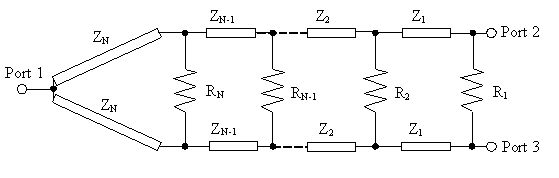The even mode analysis is done by assuming that the excitations on ports 2 and 3 are in-phase. Then the voltages across the isolation resistors are zero and they can be ignored: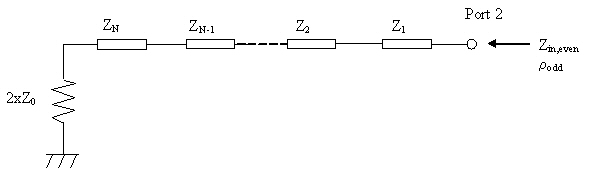In the odd mode, the excitations are 180 degrees out of phase. In this case a virtual ground lies across the axis of symmetry. The resistor values all get chopped in half: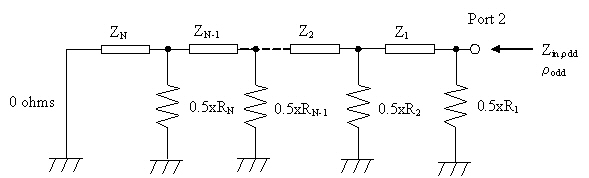From the even and odd mode schematics, the reflection coefficients can be calculated from the impedance looking in (Zin) using the transformer equation: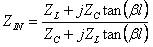Next, following Cohn's paper, we can easily arrive at the reflection coefficient at port 1, because it is the same as the even-mode reflection coefficient: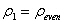The transmission coefficient (essentially S21 or S31 in S-parameters) can be calculated from the reflection coefficient at port 1 assuming that the network is lossless: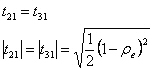The refection coefficients at ports 2 and 3 are the average value of the even and odd-mode coefficients: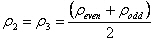And last, the isolation between ports 2 and 3 (the transmission coefficient) is equal to half of the difference between even and odd mode reflection coefficients: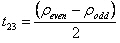Here's a few things worth pointing out:

The input (port 1) reflection coefficient is not a function of the resistors, it is merely a function of the line impedances (Z1, Z2, etc.). In other words, it behaves like a multi-section transformer, which can be solved in different ways. We chose to use Chebyshev in our spreadsheet, but it just as well could have been maximally flat.

The choice of resistors

As Mr. Cohn pointed out, this model does not take into account the effects of the sections coupling to each other, which changes even and odd mode impedances and will throw off the response a bit. If you really need first pass success, use an EM simulator for the final design.

### Examples of using the spreadsheet

The plots below used the values that Mr. Cohn developed in his paper, for seven sections. For convenience we put Cohn's computed values for his examples into our spreadsheet on a worksheet named "Cohn", you can copy them into the "Cintorl Panel" worksheet to examine the various responses he achieved. . Here's the VSWRs of the ports for a 10 GHz, seven section splitter with 164% bandwidth (F2 is 10xF1). Note the equal ripple on port 1, and the non-equal ripple on port 2 (which is because Cohn didn't have an exact way to calculate the resistors for equal ripple and neither do we!)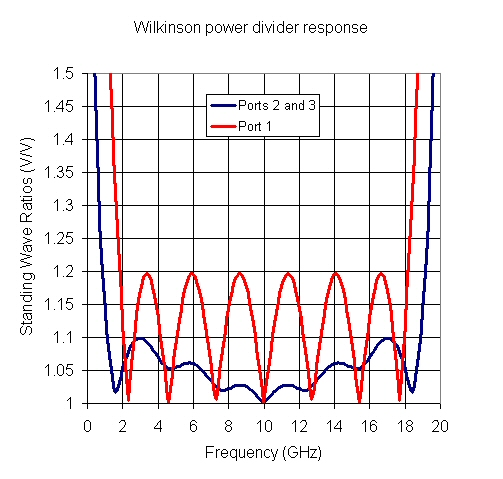Also, note that the impedance matches at ports 2 and 3 are better than the match at port 1, this is typical. Here's a plot where we converted SWRs to retun losses, if you prefer units in dB: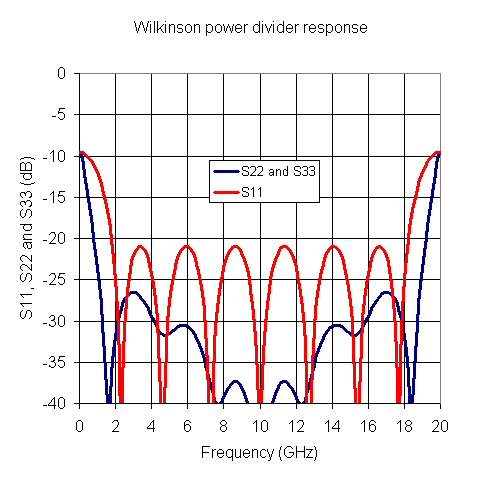Fnally, here's the isolation, which isn't exactly equal ripple either: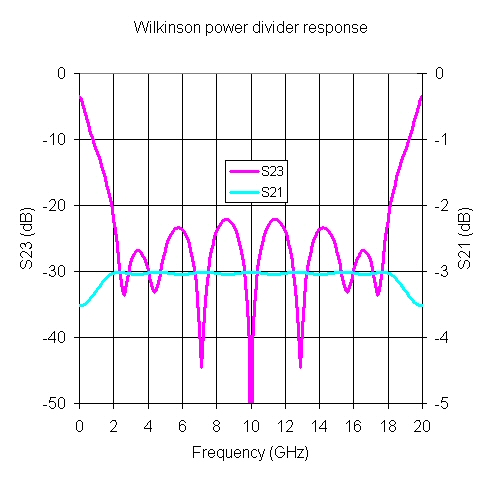Author : Unknown Editor